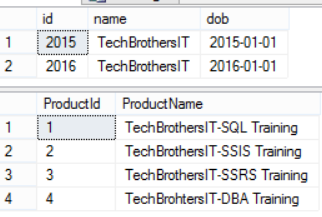C# - Import Data To Tables according to Excel Sheet Names from Excel Files dynamically in C#

You are working as C# or Dot Net Developer, You get single or multiple Excel files in one of Source Folder. The Excel file can come with single or multiple sheets. Each sheet names will be matching with the tables you have in your Database. You need to write C# program that should loop through Excel files, then Excel Sheets and load the data to tables using Sheet Name as it is equal to Table Name.

The new sheets can be added or existing sheets can be dropped anytime. C# program should be dynamic to handle the number of sheets. As long as the sheet name matches with one of our table name, it should load the data.

Here is my sample data. I have two Excel files, each contains two sheets, Product and Customer. Notice that the order of sheets does not matter. As long as the name matches with your Database tables we are good to load.How to Load multiple Sheets from Single or Multiple Excel Files to SQL Server Tables by using Sheet Name in C#

Let's create Product and Customer tables after talking a look into our Excel Sheets.

CREATE TABLE dbo.Customer (
id INT
,name VARCHAR(100)
,dob DATE
)
GO

CREATE TABLE dbo.Product (
ProductId INT
,ProductName VARCHAR(100)
)

I have created the Console application by using the below C# code to load sheets to tables.

using System;
using System.Collections.Generic;
using System.Linq;
using System.Text;
using System.IO;
using System.Data;
using System.Data.OleDb;
using System.Data.SqlClient;

namespace TechBrothersIT.com_CSharp_Tutorial
{
class Program
{
static void Main(string[] args)
{
//the datetime and Log folder will be used for error log file in case error occured
string datetime = DateTime.Now.ToString("yyyyMMddHHmmss");
string LogFolder = @"C:\Log\";
try
{
//Provide the folder path where excel files are present
String FolderPath = @"C:\Source\";
String TableName = "";
//Provide the schema for tables in which we want to load Excel files
String SchemaName = "dbo";
//Provide the Database Name in which table or view exists
string DatabaseName = "TechbrothersIT";
//Provide the SQL Server Name
string SQLServerName = "(local)";

//Create Connection to SQL Server Database to import Excel file's data
SqlConnection SQLConnection = new SqlConnection();
SQLConnection.ConnectionString = "Data Source = "
+ SQLServerName + "; Initial Catalog ="
+ DatabaseName + "; "
+ "Integrated Security=true;";

var directory = new DirectoryInfo(FolderPath);
FileInfo[] files = directory.GetFiles();

//Declare and initilize variables
string fileFullPath = "";

//Get one Book(Excel file at a time)
foreach (FileInfo file in files)
{
fileFullPath = FolderPath + "\\" + file.Name;

//Create Excel Connection
string ConStr;
string HDR;
HDR = "YES";
ConStr = "Provider=Microsoft.ACE.OLEDB.12.0;Data Source=" + fileFullPath
+ ";Extended Properties=\"Excel 12.0;HDR=" + HDR + ";IMEX=0\"";
OleDbConnection cnn = new OleDbConnection(ConStr);

//Get Sheet Name
cnn.Open();
DataTable dtSheet = cnn.GetOleDbSchemaTable(OleDbSchemaGuid.Tables, null);
string sheetname;
sheetname = "";
foreach (DataRow drSheet in dtSheet.Rows)
{
if (drSheet["TABLE_NAME"].ToString().Contains("\$"))
{
sheetname = drSheet["TABLE_NAME"].ToString();
TableName = sheetname.Replace("\$", "");

//Load the DataTable with Sheet Data so we can get the column header
OleDbCommand oconn = new OleDbCommand("select top 1 * from [" + sheetname + "]", cnn);
DataTable dt = new DataTable();
cnn.Close();

//Prepare Header columns list so we can run against Database to get matching columns for a table.
//If columns does not exists in table, it will ignore and load only matching columns data
string SQLQueryToGetMatchingColumn = "";
for (int i = 0; i < dt.Columns.Count; i++)
{
if (i != dt.Columns.Count - 1)
ExcelHeaderColumn += "'" + dt.Columns[i].ColumnName + "'" + ",";
else
ExcelHeaderColumn += "'" + dt.Columns[i].ColumnName + "'";
}

SQLQueryToGetMatchingColumn = "select STUFF((Select  ',['+Column_Name+']' from Information_schema.Columns where Table_Name='" +
TableName + "' and Table_SChema='" + SchemaName + "'" +
"and Column_Name in (" + @ExcelHeaderColumn + ") for xml path('')),1,1,'') AS ColumnList";

//Get Matching Column List from SQL Server
string SQLColumnList = "";
SqlCommand cmd = SQLConnection.CreateCommand();
cmd.CommandText = SQLQueryToGetMatchingColumn;
SQLConnection.Open();
SQLColumnList = (string)cmd.ExecuteScalar();
SQLConnection.Close();

//Use Actual Matching Columns to get data from Excel Sheet
OleDbConnection cnn1 = new OleDbConnection(ConStr);
cnn1.Open();
OleDbCommand oconn1 = new OleDbCommand("select " + SQLColumnList + " from [" + sheetname + "]", cnn1);
DataTable dt1 = new DataTable();
cnn1.Close();

//Delete the row if all values are nulll
int columnCount = dt1.Columns.Count;
for (int i = dt1.Rows.Count - 1; i >= 0; i--)
{
bool allNull = true;
for (int j = 0; j < columnCount; j++)
{
if (dt1.Rows[i][j] != DBNull.Value)
{
allNull = false;
}
}
if (allNull)
{
dt1.Rows[i].Delete();
}
}
dt1.AcceptChanges();

//Load Data from DataTable to SQL Server Table.
SQLConnection.Open();
using (SqlBulkCopy BC = new SqlBulkCopy(SQLConnection))
{
BC.DestinationTableName = SchemaName + "." + TableName;
foreach (var column in dt1.Columns)
BC.WriteToServer(dt1);
}
SQLConnection.Close();

}
}
}
}

catch (Exception exception)
{
// Create Log File for Errors
using (StreamWriter sw = File.CreateText(LogFolder
+ "\\" + "ErrorLog_" + datetime + ".log"))
{
sw.WriteLine(exception.ToString());

}

}

}
}
}

Build the program and then execute. I executed with my sample file and it loaded the Excel sheet data to tables as per names.How to import data to tables from excel sheets according to the name of sheet in C#